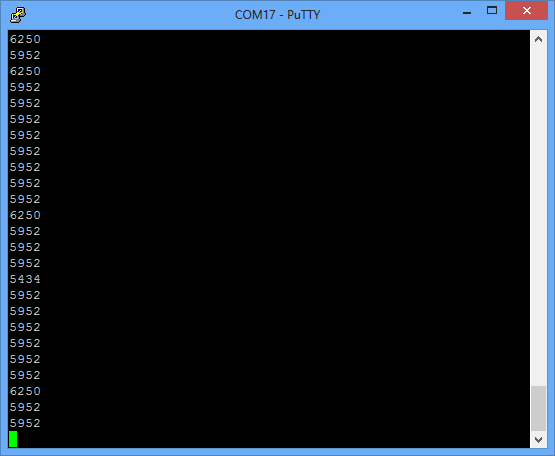# Frequency counting test

This is something I long wanted to try out – frequency counting with my Funky v2. I used Albert’s Arduino frequency counter library for the purpose. I’d test it by doing 6 kHz PWM and try to count that. The interrupt pin on Funky v2 is Digital 2 and the PWM is Digital 13, so I connected these with a jumper wire to see if I’ll get accurate readings. My code is:

```#include <FreqPeriodCounter.h>  //http://www.avdweb.nl/arduino/hardware-interfacing/frequency-period-counter.html

#define TIMER_ENABLE_PWM     (TCCR4A |= _BV(COM4A1))
#define TIMER_DISABLE_PWM    (TCCR4A &= ~(_BV(COM4A1)))
#define TIMER_ENABLE_INTR    (TIMSK4 = _BV(TOIE4))
#define TIMER_DISABLE_INTR   (TIMSK4 = 0)
#define TIMER_INTR_NAME      TIMER4_OVF_vect
#define TIMER_CONFIG_HZ(val) ({ \
const uint16_t pwmval = F_CPU / 2 / (val); \
TCCR4A = (1<<PWM4A); \
TCCR4B = _BV(CS40); \
TCCR4C = 0; \
TCCR4D = (1<<WGM40); \
TCCR4E = 0; \
TC4H = pwmval >> 8; \
OCR4C = pwmval; \
TC4H = (pwmval / 3) >> 8; \
OCR4A = (pwmval / 3) & 255; \
})

#define TIMER_PWM_PIN 13

const byte counterPin = 2;
const byte counterInterrupt = 1; // = pin 2
FreqPeriodCounter counter(counterPin, micros, 0);

void setup(void)
{ Serial.begin(9600);
attachInterrupt(counterInterrupt, counterISR, CHANGE);

TIMER_DISABLE_INTR;
pinMode(TIMER_PWM_PIN, OUTPUT);
digitalWrite(TIMER_PWM_PIN, LOW); // When not sending PWM, we want it low
TIMER_CONFIG_HZ(6000);            // Generate 6 kHz on D13, use a jumper wire to hook that to D2 where we will count it
TIMER_ENABLE_PWM;
}

void loop(void)
}

void counterISR()
{ counter.poll();
}```

and it outputs this to the serial (in Hz):As a conclusion, it works nicely, will probably come in handy in some future project.

### 2 thoughts on “Frequency counting test”

1.Peter Nolan

I’ve being interested in monitoring power grid frequency to research deviation in Ireland. Normally 50hz, I have dabbled with using emontx some while back as it counts zero crossing. This looks interesting and I have a spare Funky I got from you a while back. Any suggestions where to start to get input for Funky